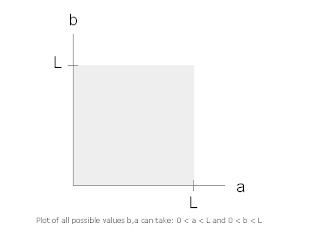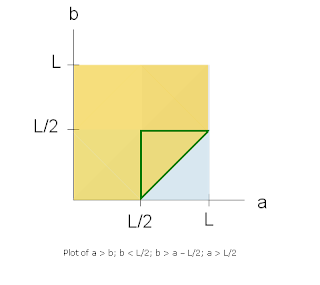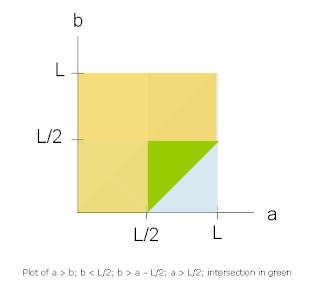## Wednesday, August 5, 2009

### Triangle Problem + Solution

Question: "If you break a straight line randomly in two places, what is the probability that you can form a triangle from the resulting three pieces?"

Please provide the proof + code for a simulation of 10,000 trails! Will post the solution tomorrow so get crackin!

------------------------------------------

Solution:

First off, congratulation are in order to a Mr. Paul R. and a Mr. Dan D. who both successfully utilized the higher power of numbers and reached the correct answer. A slow clap to you both. A special mention is in order for a Mr. Frank I. who uncovered about 80% of the solution, and to a Mr. Elliot G. who at least attempted the problem (percentage of success was not capable of being calculated for this last attempt).

The Proof. We first have to determine what, when given three different lines of varying lengths, is required to construct a Triangle? In order to create a triangle from three different lines of varying lengths, we need the following condition to hold:

Long side < short side + medium side

Now, looking at the posed question, lets say that the stick’s length is L, and we have two random breaks, a and b. We can draw the following representation:We can see that, in this case, a < b. We can also see that there are three lengths that result from this break, x, y, and z. We have the following equations now:

1. x + y + z = L
2. a < b
3. x = a
4. y = b – a
5. z = L – b

So we have to think about the value-space that a and b can take on, namely that 0 < a < L and 0 < b < L, or graphically:This is a representation of all possible values a and b can take on. Now let’s assume that x >= y >= z so from the starting condition that must be fulfilled to make a triangle, we have:

x < y + z => a < (b – a) + (L – b) => a < -a + L = a < L/2

So in order for a triangle to be the result, a must be less than half of the entire length. Furthermore, because x = a and x is the largest, y and z must also be less than half of length L. We see that no single side can be greater than or equal to L/2 if we want to be able to make a triangle from the sides.

So we can update our equations:

1. x + y + z = L
2. a < b
3. x = a and x < L/2
4. y = b – a and y < L/2
5. z = L – b and z < L/2

So we can now update our set of values drawing – we know that a < b and a < L/2 from updated equation #3, so:The values of that a and b can take on, when a < b, that will produce a triangle are now outlined in green. Next, from updated equation #5 we have that L – b < L / 2, which equals b > L / 2:The values of that a and b can take on, when a < b, that will produce a triangle are now outlined in green. Finally from updated equation #4 we have that b – a < L / 2, which equals b < a + L / 2 (this is a linear condition – line has a slope of 1 and b intercept of L/2:So the area that meets all of these conditions is our winner (in green):Which we can see is equal to 1/8 (0.125) of the possible values.

However, remember that this assumes that a < b, and because we know that a and b are both random numbers, they are interchangeable, so we can also have the following situation:So this scenario must be taken into account. We now assume a > b, which yields the following starting equations:

1. x + y + z = L
2. a > b
3. x = b and x < L/2
4. y = a – b and y < L/2
5. z = L – a and z < L/2

We are still working with the same initial work space for a and b, but we have a different limitation being applied, namely a > b.

If we rearrange all these new equations as we did above, we arrive at the following conditions:

Starting Condition: a > b
From #3: b < L / 2
From #4: b > a – L / 2
From #5: a > L / 2

Which we can plot graphically:And the only area of intersection for all equations mirrors what we found before, reflected across the line b = a:Finally, we combine both scenarios for a > b and b < a and the resulting areas that satisfy our starting stipulation (long < medium + short), and we get the following result set:Which yields and answer of 1/8 + 1/8 = 1/4 or 0.25 or 25%. So if you break a straight line randomly in two places, the probability that you can form a triangle from the resulting three pieces is 25%.

The Code. The code to run the simulation. This is written in Ruby.

#!/usr/bin/ruby
numOfTrials = 10000
numTriangles = 0

numOfTrials.times do
# generate two random breaks, ordered
breaks = [ rand, rand ].sort

# load the length calculations via the breaks into an "lengths" array
# order the lenghts
lengths = [

breaks ,
breaks - breaks ,
1 - breaks

].sort

# add 1 to triangle var if we have a triangle
numTriangles += 1 if lengths < lengths + lengths

end

pct = numTriangles.to_f / numOfTrials * 100

puts "Number of Trials: " + numOfTrials.to_s
puts "Number of Triangles: " + numTriangles.to_s
puts "Percentage: %" + pct.to_s

Running this simulation produces results that cluster around the expected value of 25%.